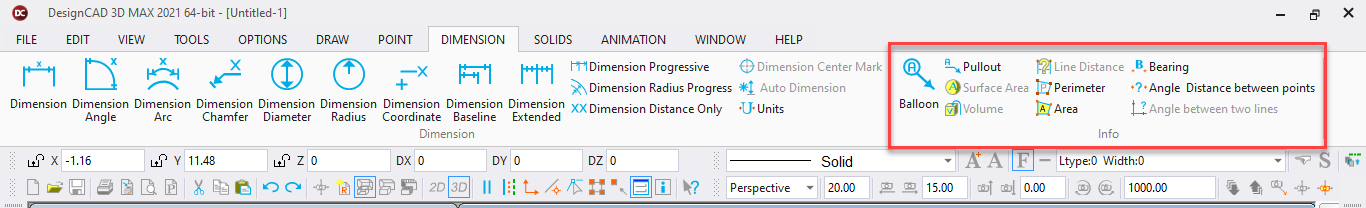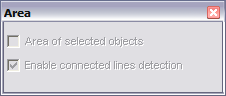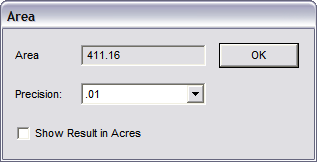# AreaCalculates the area of selected object or the area within a closed area defined by points.

Points 1-n: Determine the area to be calculated If you want to calculate the area of a selected object, select it first. Select the Area command. If an object is selected, you will see this window:Area of Selected Objects: Calculates the area of any selected objects. Overlapping regions are not subtracted from the total area. If not selected, you can select the object after choosing the Area command.

Enable Connected Lines Detection: Calculated area between selected lines that are connected.

Check this option and then check Area of Selected Objects.

If no objects are selected, just set points around the area you want to measure. The points will be connected with a temporary line. Press Enter when you have set all of the points. The area of the shape will be calculated and displayed in drawing units.Precision: Controls how many digits appear after the decimal point.

Show Results in Acres: Calculates the area in acres instead of the drawing units.